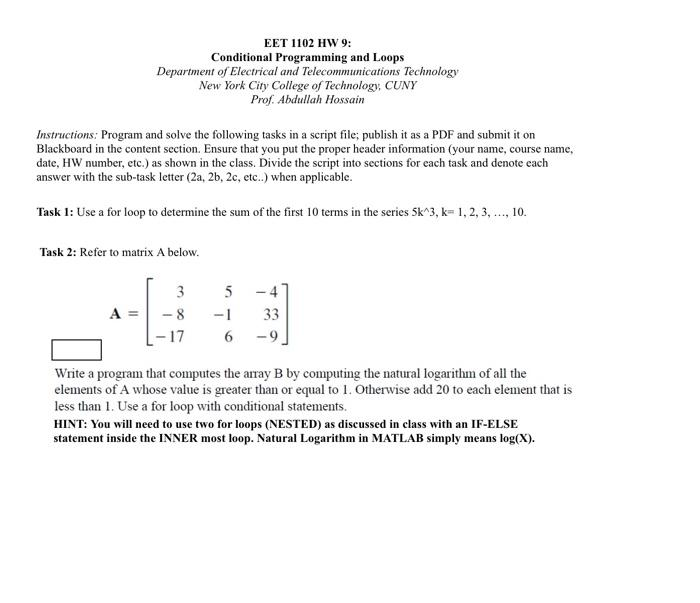Home / Expert Answers / Electrical Engineering / eet-1102-hw-9-conditional-programming-and-loops-department-of-electrical-and-telecommunications-te-pa152

# (Solved): EET 1102 HW 9: Conditional Programming and Loops Department of Electrical and Telecommunications Te ...EET 1102 HW 9: Conditional Programming and Loops Department of Electrical and Telecommunications Technology New York City College of Technology, CUNY Prof. Abdullah Hossain Instructions: Program and solve the following tasks in a script file; publish it as a PDF and submit it on Blackboard in the content section. Ensure that you put the proper header information (your name, course name, date, HW number, etc.) as shown in the class. Divide the script into sections for each task and denote each answer with the sub-task letter , etc...) when applicable. Task 1: Use a for loop to determine the sum of the first 10 terms in the series . Task 2: Refer to matrix A below. Write a program that computes the array by computing the natural logarithm of all the elements of whose value is greater than or equal to 1 . Otherwise add 20 to each element that is less than 1. Use a for loop with conditional statements. HINT: You will need to use two for loops (NESTED) as discussed in class with an IF-ELSE statement inside the INNER most loop. Natural Logarithm in MATLAB simply means .

We have an Answer from Expert

% Course Name
% Date
% Homework Number

%% Task 1: Sum of the series
sum_terms = 0;

for k = 1:10
term = 5 * k^3;
sum_terms = sum_terms + term;
end